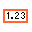# DBL Numeric Constant

Object information
Owning palette(s) Numeric palette
Type Constant
Requires Basic Development Environment
IconUse the DBL Numeric Constant to pass a double-precision, floating-point numeric value to the block diagram. Set this value by clicking inside the constant with the Operating tool and typing a value.

If you enter a floating-point number for the numeric constant, the default representation is a double-precision, floating-point number. For example, if you enter 123, the representation is a 32-bit integer.

You also can change the numeric representation of a numeric constant. If you enter an integer, the default representation is a 32-bit integer. For example, if you enter 123., the representation is a double-precision, floating-point number. If you enter a complex number, the default representation is a double-precision, complex number.

You cannot change the value of the numeric constant while the VI runs. You can assign a label to this constant.

## History

Version Change(s)More info to come.